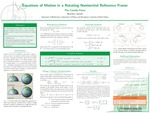## Essential Studies UNDergraduate Showcase

#### Description

Measurement is an essential part of empirical research. As such, understanding whether the frame of reference in which a measurement occurs is inertial is essential for accurate data. As a rotating sphere, Earth is a non-inertial frame of reference and gives rise to fictitious forces. These forces are derived through vector algebra and further solved through matrix differential equations. The final solution for how velocity evolves over time results in sinusoidal functions with a period of 24 hours for Earth. To test the equations further, rational scenarios are proposed for different locations on the surface of Earth involving different initial velocities, resulting in expected behavior. It is also important to demonstrate that the complete set of solutions collapse into basic kinematic equations for an inertial frame of reference under gravity when rotation of the system is absent.

Course: Math 488 – Senior Capstone

12-6-2018

Poster

Grand Forks, ND

#### Keywords

Coriolis, fictitious force, Green’s Function, non-inertial frame, coupled equations, azimuthal symmetry, matrix differential equation

#### Disciplines

Mathematics